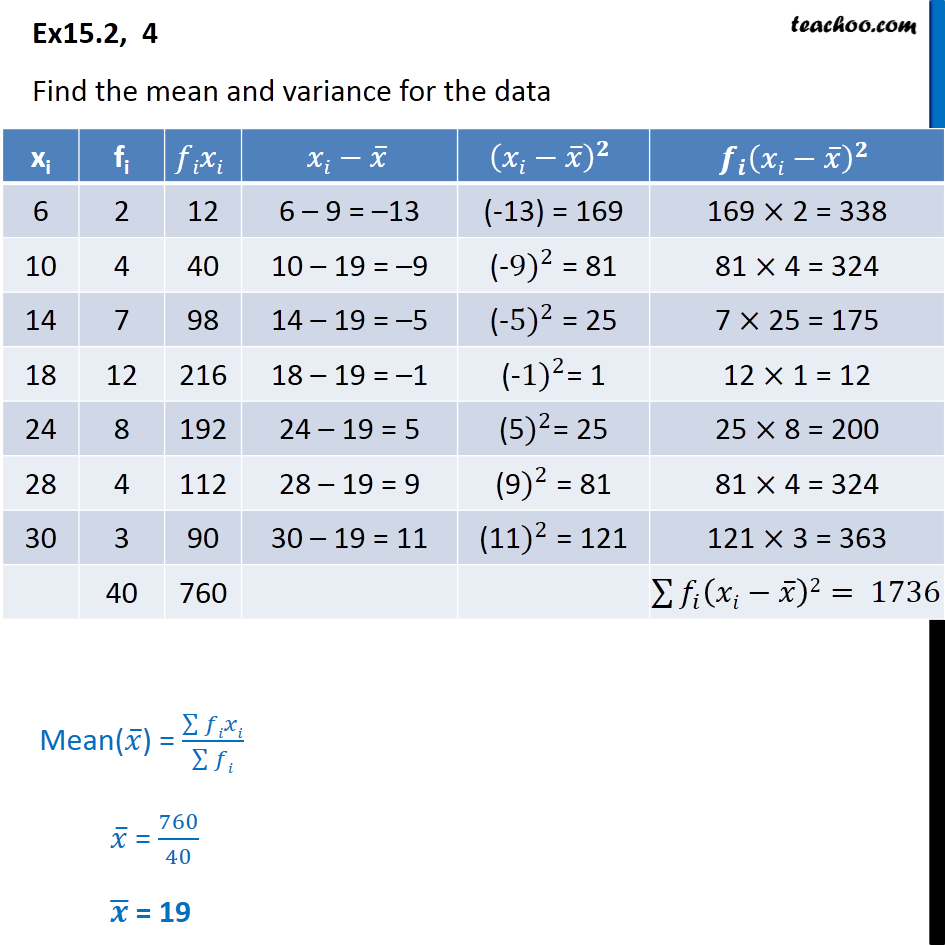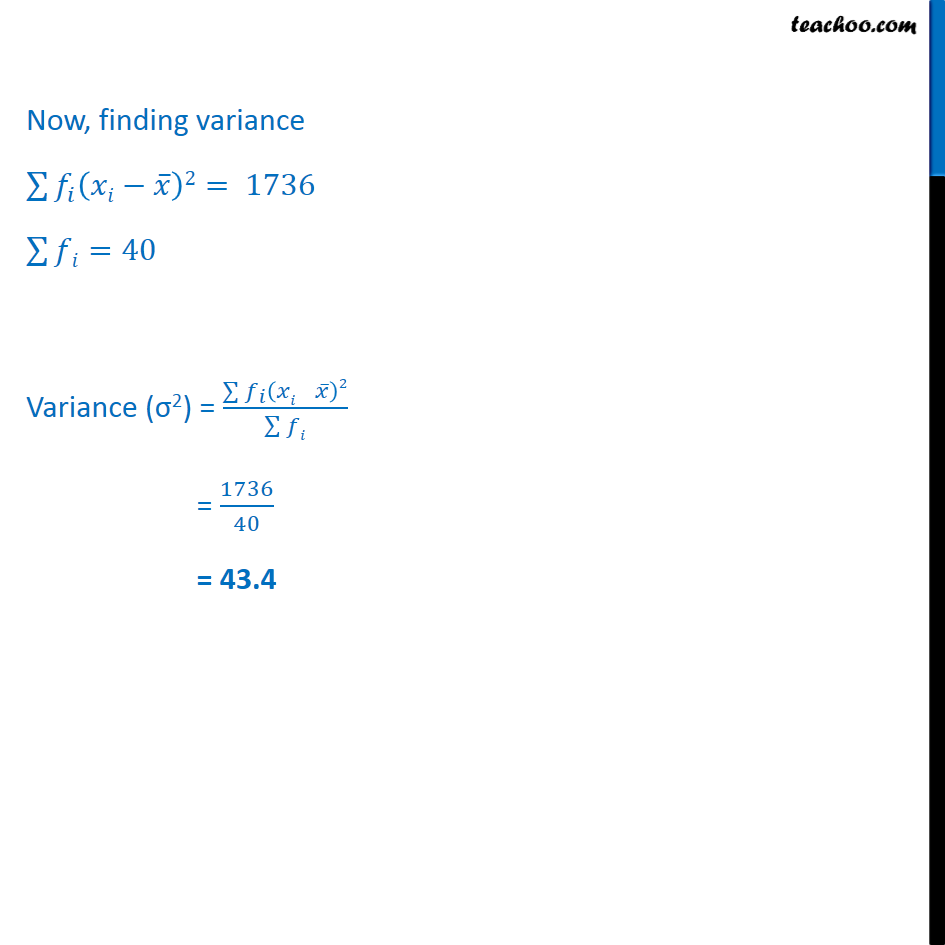Ex 13.2

Chapter 13 Class 11 Statistics
Serial order wiseLearn in your speed, with individual attention - Teachoo Maths 1-on-1 Class

### Transcript

Ex 13.2, 4 - Chapter 15 Class 11 Statistics - NCERT Find the mean and variance for the data xi fi fixi xi-x ̅ (xi-x ̅ )^2 〖f_i (xi-x ̅ )〗^2 6 2 12 6 – 9 = –13 (-13) = 169 169 × 2 = 338 10 4 40 10 – 19 = –9 (-〖9)〗^2 = 81 81 × 4 = 324 14 7 98 14 – 19 = –5 (-〖5)〗^2 = 25 7 × 25 = 175 18 12 216 18 – 19 = –1 (-〖1)〗^2= 1 12 × 1 = 12 24 8 192 24 – 19 = 5 (5)^2= 25 25 × 8 = 200 28 4 112 28 – 19 = 9 (9)^2 = 81 81 × 4 = 324 30 3 90 30 – 19 = 11 (11)^2 = 121 121 × 3 = 363 40 760 Mean(𝑥 ̅) = (∑▒𝑓𝑖𝑥𝑖)/(∑▒𝑓𝑖) 𝑥 ̅ = 760/40 𝒙 ̅ = 19 Now, finding variance fi (xi - x)^2 = 1736 =40 Variance ( 2) = ( _ ( )2 )/( ) = 1736/40 = 43.4